Note

This page is a reference documentation. It only explains the function signature, and not how to use it. Please refer to the user guide for the big picture.

# nilearn.plotting.plot_connectome#

nilearn.plotting.plot_connectome(adjacency_matrix, node_coords, node_color='auto', node_size=50, edge_cmap=<matplotlib.colors.LinearSegmentedColormap object>, edge_vmin=None, edge_vmax=None, edge_threshold=None, output_file=None, display_mode='ortho', figure=None, axes=None, title=None, annotate=True, black_bg=False, alpha=0.7, edge_kwargs=None, node_kwargs=None, colorbar=False, radiological=False)[source]#

Plot connectome on top of the brain glass schematics.

The plotted image should be in MNI space for this function to work properly.

In the case of ‘l’ and ‘r’ directions (for hemispheric projections), markers in the coordinate x == 0 are included in both hemispheres.

Parameters:
adjacency_matrixnumpy array of shape (n, n)

Represents the link strengths of the graph. The matrix can be symmetric which will result in an undirected graph, or not symmetric which will result in a directed graph.

node_coordsnumpy array_like of shape (n, 3)

3d coordinates of the graph nodes in world space.

node_colorcolor or sequence of colors or ‘auto’, optional

Color(s) of the nodes. If string is given, all nodes are plotted with same color given in string.

node_sizescalar or array_like, optional

Size(s) of the nodes in points^2. Default=50.

edge_cmapcolormap, optional

Colormap used for representing the strength of the edges. Default=cm.bwr.

edge_vmin, edge_vmaxfloat, optional

If not None, either or both of these values will be used to as the minimum and maximum values to color edges. If None are supplied the maximum absolute value within the given threshold will be used as minimum (multiplied by -1) and maximum coloring levels.

edge_thresholdstr or number, optional

If it is a number only the edges with a value greater than edge_threshold will be shown. If it is a string it must finish with a percent sign, e.g. “25.3%”, and only the edges with a abs(value) above the given percentile will be shown.

output_file`str`, or None, optional

The name of an image file to export the plot to. Valid extensions are .png, .pdf, .svg. If output_file is not None, the plot is saved to a file, and the display is closed.

display_modestring, optional

Choose the direction of the cuts: ‘x’ - sagittal, ‘y’ - coronal, ‘z’ - axial, ‘l’ - sagittal left hemisphere only, ‘r’ - sagittal right hemisphere only, ‘ortho’ - three cuts are performed in orthogonal directions. Possible values are: ‘ortho’, ‘x’, ‘y’, ‘z’, ‘xz’, ‘yx’, ‘yz’, ‘l’, ‘r’, ‘lr’, ‘lzr’, ‘lyr’, ‘lzry’, ‘lyrz’. Default=’ortho’.

figure`int`, or `matplotlib.figure.Figure`, or None, optional

Matplotlib figure used or its number. If None is given, a new figure is created.

axes`matplotlib.axes.Axes`, or 4 tupleof `float`: (xmin, ymin, width, height), default=None

The axes, or the coordinates, in matplotlib figure space, of the axes used to display the plot. If None, the complete figure is used.

title`str`, or None, default=None

The title displayed on the figure.

annotate`bool`, default=True

If annotate is True, positions and left/right annotation are added to the plot.

black_bg`bool`, or “auto”, optional

If True, the background of the image is set to be black. If you wish to save figures with a black background, you will need to pass facecolor=”k”, edgecolor=”k” to `matplotlib.pyplot.savefig`. Default=False.

alphafloat between 0 and 1, optional

Alpha transparency for the brain schematics. Default=0.7.

edge_kwargsdict, optional

Will be passed as kwargs for each edge matlotlib Line2D.

node_kwargsdict, optional

Will be passed as kwargs to the plt.scatter call that plots all the nodes in one go.

colorbar`bool`, optional

If True, display a colorbar on the right of the plots. Default=False.

radiological`bool`, default=False

Invert x axis and R L labels to plot sections as a radiological view. If False (default), the left hemisphere is on the left of a coronal image. If True, left hemisphere is on the right.

`nilearn.plotting.find_parcellation_cut_coords`

Extraction of node coords on brain parcellations.

`nilearn.plotting.find_probabilistic_atlas_cut_coords`

Extraction of node coords on brain probabilistic atlases.

## Examples using `nilearn.plotting.plot_connectome`#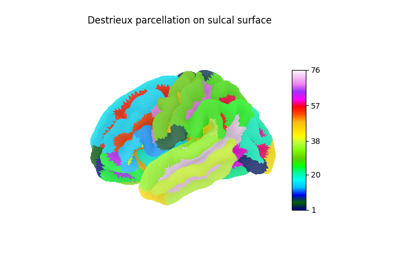Computing a connectome with sparse inverse covariance

Computing a connectome with sparse inverse covariance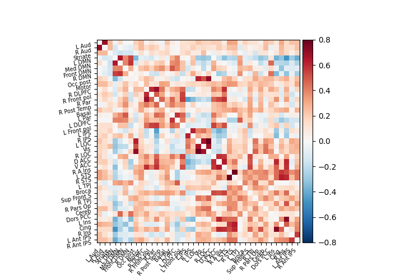Extracting signals of a probabilistic atlas of functional regions

Extracting signals of a probabilistic atlas of functional regions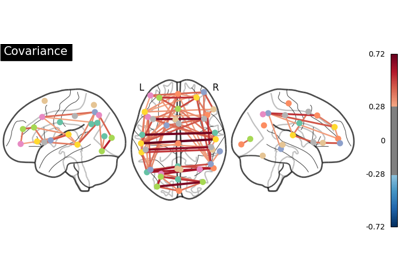Group Sparse inverse covariance for multi-subject connectome

Group Sparse inverse covariance for multi-subject connectome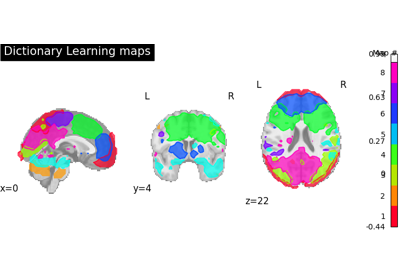Regions extraction using dictionary learning and functional connectomes

Regions extraction using dictionary learning and functional connectomes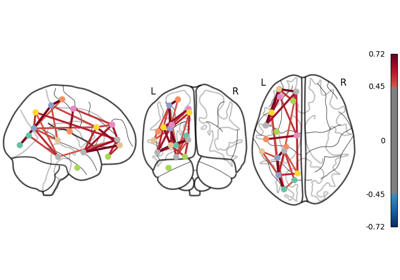Comparing connectomes on different reference atlases

Comparing connectomes on different reference atlases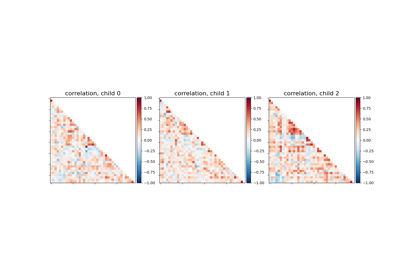Classification of age groups using functional connectivity

Classification of age groups using functional connectivity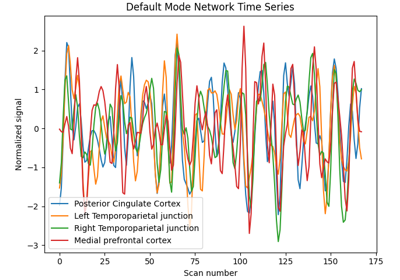Extract signals on spheres and plot a connectome

Extract signals on spheres and plot a connectome